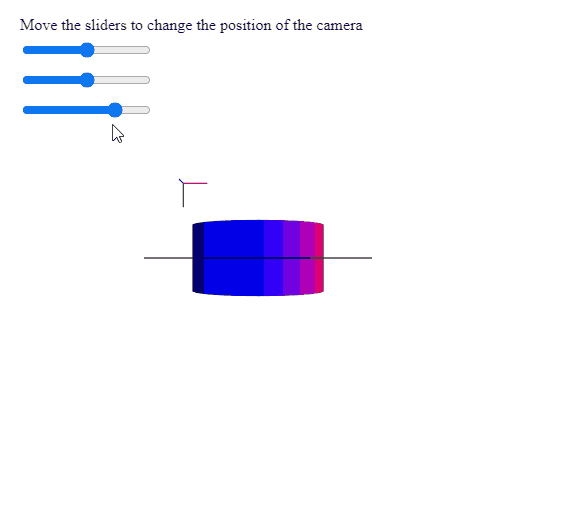# p5.Camera setPosition() Method

• Last Updated : 28 Jul, 2020

The setPosition() method of p5.Camera in p5.js is used to set the position of the camera to the given point in world space. It maintains the current camera orientation while changing the position.

Syntax:

Hey geek! The constant emerging technologies in the world of web development always keeps the excitement for this subject through the roof. But before you tackle the big projects, we suggest you start by learning the basics. Kickstart your web development journey by learning JS concepts with our JavaScript Course. Now at it's lowest price ever!

```setPosition( x, y, z )
```

Parameters: This method accepts three parameters as mentioned above and described below:

• x: It is a number that denotes the x position of the point in world space.
• y: It is a number that denotes the y position of the point in world space.
• z: It is a number that denotes the z position of the point in world space.

The example below illustrates the setPosition() method in p5.js:

Example:

## Javascript

 `let currCamera;`` ` `function` `setup() {``  ``createCanvas(500, 500, WEBGL);``  ``helpText = createP(``    ``"Move the sliders to change the "` `+``    ``"position of the camera"``  ``);``  ``helpText.position(20, 0);`` ` `  ``// Create the camera``  ``currCamera = createCamera();`` ` `  ``// Create three sliders for changing the``  ``// position of the camera``  ``xPosSlider = createSlider(-360, 360, 0);``  ``xPosSlider.position(20, 40);`` ` `  ``yPosSlider = createSlider(-360, 360, 0);``  ``yPosSlider.position(20, 70);`` ` `  ``zPosSlider = createSlider(0, 800, 600);``  ``zPosSlider.position(20, 100);``}`` ` `function` `draw() {``  ``clear();``  ``lights();``  ``normalMaterial();``  ``debugMode();`` ` `  ``// Get the x, y, z values from the``  ``// sliders``  ``let currX = xPosSlider.value();``  ``let currY = yPosSlider.value();``  ``let currZ = zPosSlider.value();`` ` `  ``// Set the position of the camera``  ``// to these points in the world space``  ``currCamera.setPosition(currX, currY, currZ);`` ` `  ``cylinder(90);``}`

Output:Online editor: https://editor.p5js.org/

My Personal Notes arrow_drop_up